The Octagons ClipArt gallery contains 20 illustrations of closed two-dimensional geometric figures with eight sides. Images include examples of regular (those having 8 equal sides and 8 equal angles), irregular, concentric, concave, and convex octagons.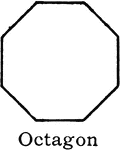### Octagon

"A polygon of...eight sides [is] an octagon." —Hallock 1905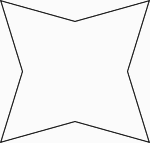### Concave Equilateral Octagon

Illustration of a concave equilateral octagon.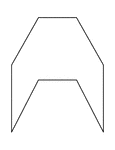### Irregular Concave Octagon

Illustration of an irregular concave octagon. This polygon has some symmetry.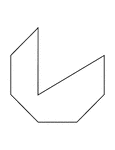### Irregular Concave Octagon

Illustration of an irregular concave octagon.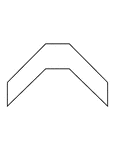### Irregular Concave Octagon

Illustration of an irregular concave octagon. This polygon has some symmetry.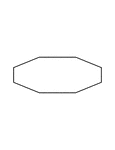### Irregular Convex Octagon

Illustration of an irregular convex octagon. This polygon has some symmetry.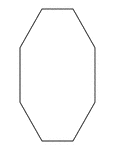### Irregular Convex Octagon

Illustration of an irregular convex octagon. This polygon has some symmetry.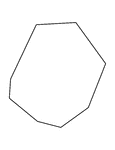### Irregular Convex Octagon

Illustration of an irregular convex octagon.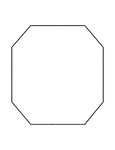### Irregular Convex Octagon

Illustration of an irregular convex octagon. This polygon has some symmetry.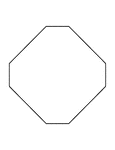### Irregular Convex Octagon

Illustration of an irregular convex octagon. This polygon has some symmetry.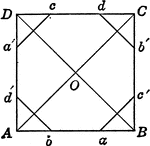### Construction of Octagon From Square

Octagon constructed from a square.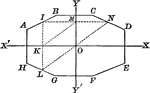### Octagon with Symmetry

Illustration of an octagon with symmetry with respect to two axes perpendicular to each other. It is…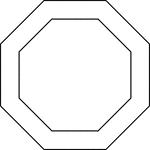### 2 Concentric Octagons

Illustration of 2 regular concentric octagons.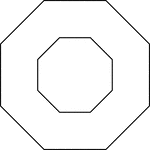### 2 Concentric Octagons

Illustration of 2 regular concentric octagons.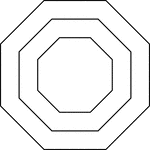### 3 Concentric Octagons

Illustration of 3 regular concentric octagons that are equally spaced.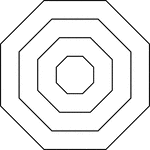### 4 Concentric Octagons

Illustration of 4 regular concentric octagons that are equally spaced.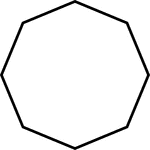### 8-sided Polygon

Polygon consisting of 8 sides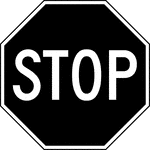### Stop, Black and White

This sign indicates that vehicles must come to a complete stop. It is to be installed at the right side…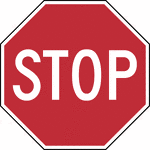### Stop, Color

This sign indicates that vehicles must come to a complete stop. It is to be installed at the right side…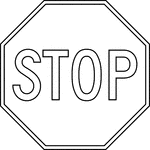### Stop, Outline

This sign indicates that vehicles must come to a complete stop. It is to be installed at the right side…